This is “Bonds with Other Than Annual Interest Payments”, section 14.6 from the book Business Accounting (v. 2.0). For details on it (including licensing), click here.

For more information on the source of this book, or why it is available for free, please see the project's home page. You can browse or download additional books there. To download a .zip file containing this book to use offline, simply click here.

Has this book helped you? Consider passing it on:
Creative Commons supports free culture from music to education. Their licenses helped make this book available to you.
DonorsChoose.org helps people like you help teachers fund their classroom projects, from art supplies to books to calculators.

## 14.6 Bonds with Other Than Annual Interest Payments

### Learning Objectives

At the end of this section, students should be able to meet the following objectives:

1. Realize that cash interest payments are often made more frequently than once a year, such as each quarter or semiannually.
2. Determine the stated interest rate, the effective interest rate, and the number of time periods to be used in a present value computation when interest payments cover a period of time of less than a year.
3. Compute the stated cash interest payments and the effective interest rate when interest is paid on a bond more frequently than once each year.
4. Prepare journal entries for a bond when the interest payments are made for a period of time shorter than a year.

Question: In the previous examples, both the interest rates and payments always covered a full year. How is this process affected if interest payments are made at other time intervals such as each quarter or semiannually?

As an illustration, assume that on January 1, Year One, an entity issues term bonds with a face value of \$500,000 that will come due in six years. Cash interest payments at a 6 percent annual rate are required by the contract. However, the actual disbursements are made every six months on June 30 and December 31. In setting a price for these bonds, the debtor and the creditor negotiate an effective interest rate of 8 percent per year. How is the price of a bond determined and the debt reported if interest payments occur more often than once each year?

Answer: None of the five basic steps for issuing and reporting a bond is affected by a change in the frequency of interest payments. However, both the stated cash rate and the effective rate must be set to agree with the time interval between payment dates. The number of time periods used in the present value computation also varies based on the time that passes from one payment to the next.

In this current example, interest is paid semiannually, so each time period is only six months in length. The stated cash interest rate to be paid during that period is 3 percent or 6/12 of the annual 6 percent rate listed in the bond contract. Similarly, the effective interest rate is 4 percent or 6/12 of the annual 8 percent negotiated rate. Both of these interest rates must align with the specific amount of time between payments. Over the six years until maturity, the bond is outstanding for twelve of these six-month periods of time.

Thus, for this bond, the cash flows will be the interest payments followed by settlement of the face value.

• Interest Payments: \$500,000 face value times 3 percent stated rate for a \$15,000 interest payment every six months during these twelve periods. Equal payments are made at equal time intervals making this an annuity. Because payments are made at the end of each period, these payments constitute an ordinary annuity.
• Face value: \$500,000 is paid at the end of these same twelve periods. This cash payment is a single amount.

As indicated, the effective rate to be used in determining the present value of these cash payments is 4 percent per period or 6/12 times 8 percent.

### Present Value of Annuity Due of \$1

http://www.principlesofaccounting.com/ART/fv.pv.tables/pvof1.htm

### Present Value of Ordinary Annuity of \$1

http://www.principlesofaccounting.com/ART/fv.pv.tables/pvofordinaryannuity.htm

• The present value of \$1 in twelve periods at an effective rate of 4 percent per period is \$0.62460.
• The present value of an ordinary annuity of \$1 for twelve periods at an effective rate of 4 percent per period is \$9.38507.
• The present value of the face value cash payment is \$500,000 times \$0.62460 or \$312,300.
• The present value of the cash interest payments every six months is \$15,000 times \$9.38507 or \$140,776 (rounded).
• The total present value of the cash flows established by this contract is \$312,300 plus \$140,776 or \$453,076. As shown in Figure 14.24 "January 1, Year One—Issuance of \$500,000 Bond with a 3 Percent Stated Rate to Yield Effective Rate of 4 Percent Semiannually", the bond is issued for this present value amount. With this payment, the agreed-upon effective rate of interest (8 percent for a full year or 4 percent for each six-month period) will be earned by the investor over the entire life of the bond.

Figure 14.24 January 1, Year One—Issuance of \$500,000 Bond with a 3 Percent Stated Rate to Yield Effective Rate of 4 Percent Semiannually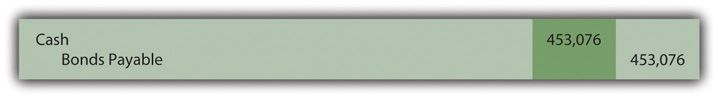On June 30, Year One, the first \$15,000 interest payment is made as reported in Figure 14.25 "June 30, Year One—Cash Interest Paid on Bond for Six-Month Period". However, the effective interest rate for that period is the principal of \$453,076 times the six-month negotiated rate of 4 percent or \$18,123 (rounded). Therefore, the interest to be compounded for this first six-month period is \$3,123 (\$18,123 interest less \$15,000 payment). That is the amount of interest recognized but not yet paid that is added to the liability as shown in Figure 14.26 "June 30, Year One—Interest on Bond Adjusted to Effective Rate".

Figure 14.25 June 30, Year One—Cash Interest Paid on Bond for Six-Month Period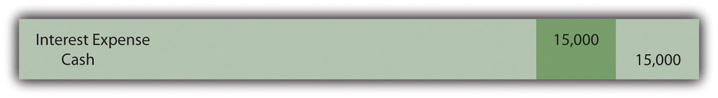Figure 14.26 June 30, Year One—Interest on Bond Adjusted to Effective Rate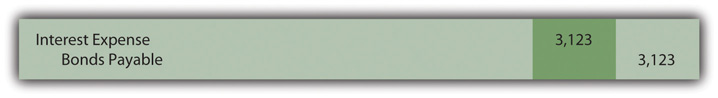The compound interest recorded previously raises the bond’s principal to \$456,199 (\$453,076 principal plus \$3,123 in compound interest). The principal is gradually moving to the \$500,000 face value. Another \$15,000 in cash interest is paid on December 31, Year One (Figure 14.27 "December 31, Year One—Cash Interest Paid on Bond for Six-Month Period"). The effective interest for this second six-month period is \$18,248 (rounded) or \$456,199 times 4 percent interest. The compound interest recognized on December 31, Year One, is \$3,248 (\$18,248 less \$15,000), a balance that is recognized by the entry presented in Figure 14.28 "December 31, Year One—Interest on Bond Adjusted to Effective Rate".

Figure 14.27 December 31, Year One—Cash Interest Paid on Bond for Six-Month Period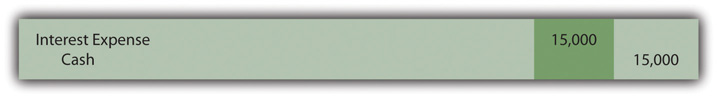Figure 14.28 December 31, Year One—Interest on Bond Adjusted to Effective Rate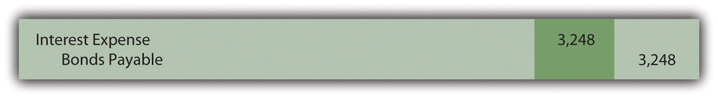The Year One income statement will report interest expense of \$18,123 for the first six months and \$18,248 for the second, giving a total for that year of \$36,371. The second amount is larger than the first because of compounding.

The December 31, Year One, balance sheet reports the bond payable as a noncurrent liability of \$459,447. That is the original principal (present value) of \$453,076 plus compound interest of \$3,123 (first six months) and \$3,248 (second six months). Once again, interest each period has been adjusted from the cash rate stated in the bond contract to the effective rate negotiated by the two parties. Here, the annual rates had to be halved because payments were made semiannually.

### Test Yourself

Question:

Friday Corporation issues a two-year bond on January 1, Year One, with a \$300,000 face value and a stated annual cash rate of 8 percent. The bond was sold to earn an effective annual rate of 12 percent. Interest payments are made quarterly beginning on April 1, Year One. The present value of \$1 at a 3 percent rate in eight periods is \$0.78941. The present value of \$1 at a 12 percent rate in two periods is \$0.79719. The present value of an ordinary annuity of \$1 at a 3 percent rate for eight periods is \$7.01969. The present value of an ordinary annuity of \$1 at a 12 percent rate for two periods is \$1.69005. What amount will Friday receive for this bond?

1. \$276,055
2. \$278,941
3. \$284,785
4. \$285,179

Answer:

The correct answer is choice b: \$278,941.

Explanation:

This bond pays interest of \$6,000 every three months (\$300,000 × 8 percent × 3/12) and \$300,000 in two years. Based on quarterly payments, effective interest is 3 percent (12 percent × 3/12 year) and cash flows are for eight periods (every three months for two years). Present value of the interest is \$6,000 × \$7.01969, or \$42,118. Present value of the face value is \$300,000 × \$0.78941, or \$236,823. Total present value (price of the bond) is \$42,118 + \$236,823, or \$278,941.

### Key Takeaway

Bonds often pay interest more frequently than once a year—for example, at an interval such as every three months or six months. If the stated cash rate and the effective rate differ, determination of present value is still required to arrive at the principal amount to be paid when the bond is issued. However, the present value computation must be adjusted to reflect the change in the length of a time period. The amount of time between payments is considered one period. The stated cash interest rate, the effective rate negotiated by the parties, and the number of time periods until maturity are all set for that particular time. The actual accounting and reporting are not affected, merely the method by which the interest rates and the number of periods are calculated.

### Talking With a Real Investing Pro (Continued)

Following is a continuation of our interview with Kevin G. Burns.

Question: Assume that you are investigating two similar companies. You are thinking about recommending one of them to your clients as an investment possibility. The financial statements look much the same except that one of these companies has an especially low amount of noncurrent liabilities whereas the other has a noncurrent liability total that seems quite high. How does that difference impact your thinking? Long-term liabilities are a great way to gain financing because a company can make use of someone else’s money. However, debt does increase risk.

Kevin Burns: I have managed to do well now for many years by being a conservative investor. My preference is always for the company that is debt free or as close to debt free as possible. I do not like financial leverage, never have. I even paid off my own home mortgage more than ten years ago.

On the other hand, long-term liabilities have to be analyzed as they are so very common. Is any of the debt convertible into capital stock so that it could potentially dilute everyone’s ownership in the company? Is the company forced to pay a high rate of interest? Why was the debt issued? In other words, how did the company use all that cash that it received? As with virtually every area of financial reporting, you have to look behind the numbers to see what is actually happening. That is why transparency is important. If the debt was issued at a low interest rate in order to make a smart acquisition, I am impressed. If the debt has a high rate of interest and the money was not well used, that is not attractive to me at all.

### Video Clip

(click to see video)

Professor Joe Hoyle talks about the five most important points in Chapter 14 "In a Set of Financial Statements, What Information Is Conveyed about Noncurrent Liabilities Such as Bonds?".